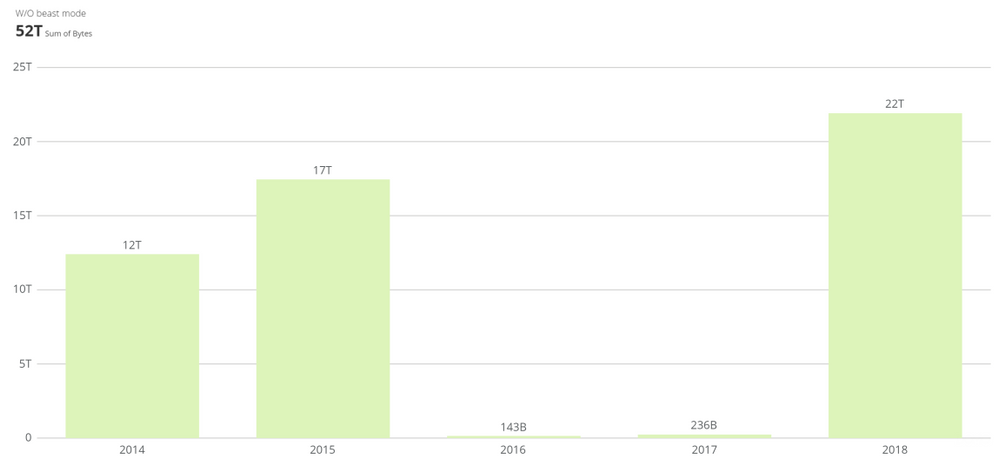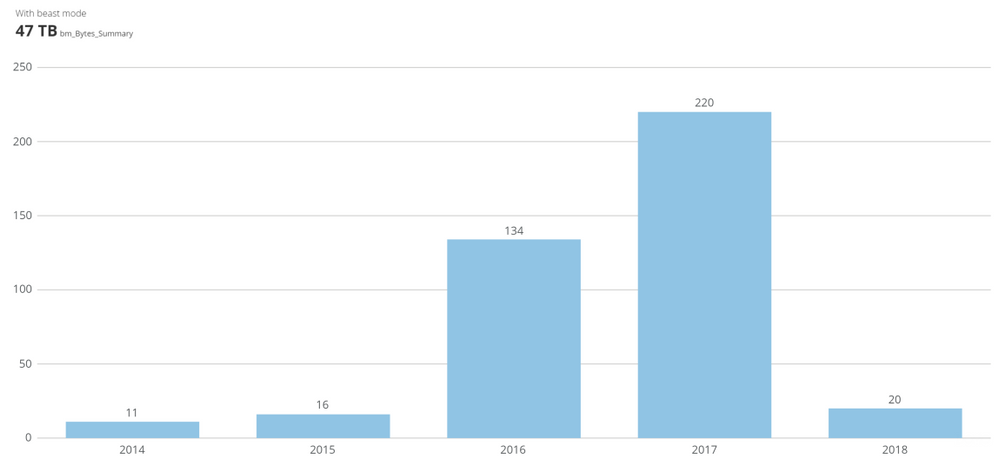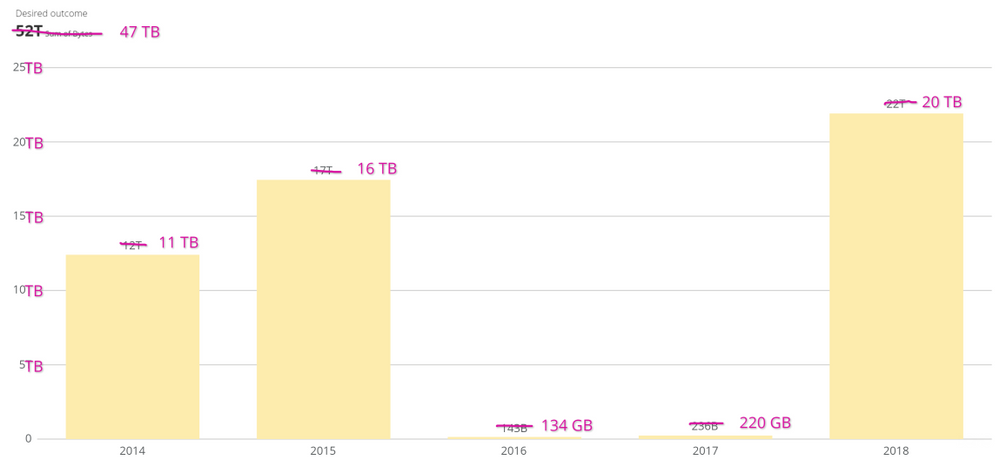# How to abbreviate numbers as KB, MB, GB, TB

I am building a card to display storage disk capacity. The raw numbers are in bytes, and I would like to abbreviate the numbers as KB, MB, GB, or TB. I would like to use the binary instead of the decimal system to abbreviate numbers in the charts. Any option how can I do this in Domo.

• Hi there,

I am out of my league in completely understanding the impact of your requirement for binary, but I would restate your volumes of bytes using a Beast Mode that calculates and concatenates.

--------------------------------------

CASE

WHEN 'Bytes' < 1048576 THEN concat(round( 'Bytes' / 1024,0),' KB')

WHEN 'Bytes' <1073741824 THEN concat(round( 'Bytes' /1048576,0),' MB')

WHEN 'Bytes' <1099511627776 THEN concat(round( 'Bytes' /1073741824 ,0),' GB')

ELSE concat(round( 'Bytes' /1099511627776,0),' TB')

END

---------------------------------------

Based on

• 1 Kilobyte (K / KB) = 2^10 bytes = 1,024 bytes
• 1 Megabyte (M / MB) = 2^20 bytes = 1,048,576 bytes
• 1 Gigabyte (G / GB) = 2^30 bytes = 1,073,741,824 bytes
• 1 Terabyte (T / TB) = 2^40 bytes = 1,099,511,627,776 bytes
Domo Consultant

**Say "Thanks" by clicking the "heart" in the post that helped you.
**Please mark the post that solves your problem by clicking on "Accept as Solution"
• Hi @Chips,

Thank you for the attention. Not quite yet the solution.

I want to find if there is an alternative to the default scale abbreviation settings. I would like that Domo abreviates the data labels using the binary scale instead. Let me attemp to explain further.

Lets imagine this is the raw data:

 Year Bytes 2014 12,404,541,416,472 2015 17,448,001,114,808 2016 143,395,525,424 2017 236,469,280,536 2018 21,913,364,667,932

Analyzing the data with a single bar and applying the default scale abbreviation, you can see the data labels follow the default decimal scale abbreviation. (e.g. T:Trillion, B:Billion)Domo uses the decimal scale by default (e.g. T: Trillion, B:Billion, etc)

Applying the beast you shared, I created two variables. A string that concatenates the value and symbol, and also a numeric that calculates just the value in the proper binary scale. I had to do that because I cannot use directly the string in the "Y axis" as it just allows me to count.

Here is the output using the beast mode. The summary number is perfect, but the data labels are not correct as scales are mixed. (e.g. Terabytes and Gigabytes in the same chart)Values were transformed to the proper binary scale. However, the chart is not representing them correctly as scales are mixed (2014-15&18 is Terabyte and 2016-17 are in Gigabyte)

What I am looking for is a way to ask domo abbreviates the data labels using the binary scale as in the below picture:Desired data label scale abbreviation (Using binary scale)

This discussion has been closed.